# ISEE Lower Level Math : How to find the perimeter of a square

## Example Questions

### Example Question #11 : How To Find The Perimeter Of A Square

Which of the following is the perimeter of a square that has an area of 9 square inches?

Possible Answers:

14 inches

9 inches

12 inches

6 inches

Correct answer:

12 inches

Explanation:

If a square has an area of 9 square inches, each side is 3 inches. This is because 3 inches times 3 inches is 9 inches. (The formula for the area of a square is length times hieght.)

Given that a square has 4 sides, we get the perimeter by multiplying 3 by 4, giving us a perimeter of 12 inches.

### Example Question #11 : Squares

Debby has a square piece of paper that has an area of 36. What is its perimeter?

Possible Answers:Correct answer:Explanation:

For a square, all four sides are equal, and the area is calculated by multiplying one side by itself.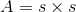Since the area of the square is 36, we know that one side would be equal to 6 because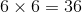.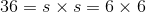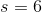Since each side is 6, the perimeter would be calculated by adding these four sides together.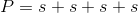This would give us: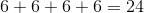Or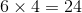### Example Question #12 : Squares

If the length of one side of a square is equal to, what is the value of the perimeter?

Possible Answers: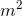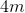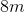None of these

Correct answer:Explanation:

The perimeter of a square is equal to the sum of all of its sides.If a side is, that means the perimeter would be equal to a value of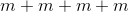, which is the equivalent of; the correct answer.

### Example Question #13 : Squares

Billy built a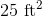enclosure for his puppy in his backyard, in the shape of a square. One side consisted of the wall of his home, while the other three sides consisted of a fence that he built. How many feet of fence did Billy build?

Possible Answers: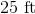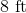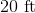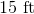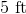Correct answer:Explanation:

The area of a square is the product of one side times another side. Since the sides of a square are equal lengths, this is equal to: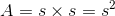Given that the area was 25 square feet, one side would be the square root of 25 (given that all sides of a square are equaly).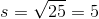The square root of 25 is 5, which would be the length of one side. Since one side of the enclosure is the wall of Billy's home, Billy only had to build 3 additional sides, each of length 5.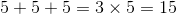Since 3 times 5 is 15, the correct answer is 15.

### Example Question #14 : Squares

If a square has an area of 100 square feet, what is the perimeter?

Possible Answers: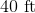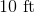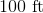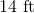Correct answer:Explanation:

When you have the area of a square, you can deduce the length of the sides by taking the square root of the area.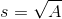Given that the area is 100 square feet, and that the square root of 100 is 10, each side is 10 feet.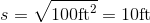With 4 sides, that means that the perimeter of a square would be 4 times 10, which is 40.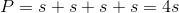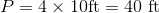### Example Question #15 : Squares

If the area of a square is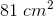, what is the perimeter?

Possible Answers: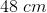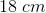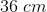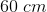Correct answer:Explanation:

The area of a square is given by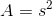, whereis the sidelength.

Plug in the given information: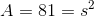Take the square root of both sides: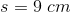The perimeter of a square is given by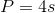, whereis again the sidelength.

Plug in the sidelength that we just calculated: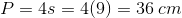### Example Question #16 : Squares

If the area of a square is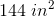, what is the perimeter?

Possible Answers: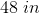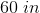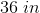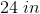Correct answer:Explanation:

The area of a square is given by, whereis the sidelength.

Plug in the perimeter given in the question: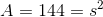Take the square root of both sides: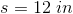The perimeter of a square is given by, whereis again the sidelength.

Plug the sidelength we just calculated into the perimeter formula: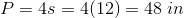### Example Question #12 : How To Find The Perimeter Of A Square

What type of a shape always has 4 equal sides?

Possible Answers:

Rhombus

Polygon

Square or rhombus

Quadrilateral

Square

Correct answer:

Square or rhombus

Explanation:

Squares, rhombuses, rectangles, and quadrilaterals all have four sides, but only squares and rhombuses have four equal sides.A square also has four equal angles (right angles), while a rhombus has two pairs of congruent angles.

A rectangle has four equal angles and two pairs of congruent sides. A quadrilateral refers to any four-sided shape.

### Example Question #17 : How To Find The Perimeter Of A Square

If the area of a square is 81, what is the perimeter?

Possible Answers:Correct answer:Explanation:

If the area of a square is 81, the length of one side can be found by taking the square root. Given that the square root of 81 is 9, the length of a side of the square is 9.

The perimeter is the sum of all four sides. Therefore, the perimeter is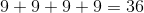.

### Example Question #18 : How To Find The Perimeter Of A Square

Find the perimeter of a square whose side length is.

Possible Answers:Correct answer:Explanation:

To solve, simply use the formula for the perimeter of a square. Thus,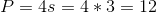### All ISEE Lower Level Math Resources